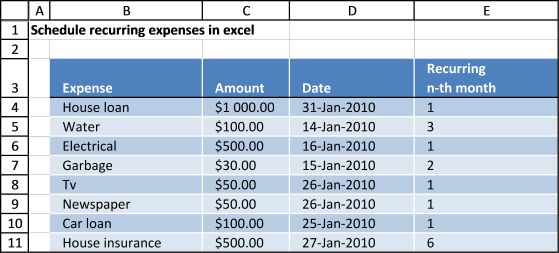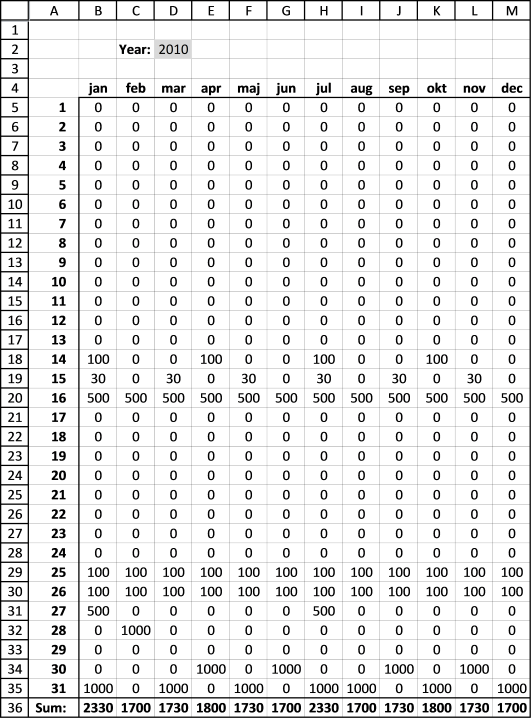Author: Oscar Cronquist Article last updated on November 18, 2016

Below is an excel table containing recurring expenses and corresponding amounts, dates and recurring intervals. An excel table allows you to easily add/delete records without changing the formulas, in other words cell refs to the table are dynamic.I am going to use this data set and create a calendar with each expense at the correct date (and recurring dates).The data above is also dynamic, if you change the year in cell D2 formulas in cell range B5:M35 are recalculated.

Array formula in B5:

=SUM(IF(DATE(\$D\$2, MONTH(B\$4), \$A5)=EDATE(TRANSPOSE(Table1[Date]), (ROW(\$1:\$1000)-1)*TRANSPOSE(Table1[Recurring
n-th month])), TRANSPOSE(Table1[Amount]), ""))

How to create an array formula

1. Type the formula in the formula bar
2. Press and hold CTRL + SHIFT
3. Press Enter once

If you made it right the formula is now surrounded by curly brackets, like this: {=array_formula} in the formula bar.

Copy formula

Copy cell B5 and paste it down to cell B35.

Copy cell range B5:B35 and paste it to the right all the way to M5:M35.

Formula in B4:

=DATE(\$D\$2, COLUMN(A1), 1) + ENTER.

Copy cell B4 and paste it right to cell M4.

Formula in B36:

=SUM(B5:B35) + ENTER.

Copy cell B36 and paste it to the right to cell M36.

### Explaining array formula in cell B5

Step 1 - Calculate date in cell B5

DATE(\$D\$2,MONTH(B\$4),\$A5)

becomes

DATE(2010,1,1)

and returns 2010-1-1 (1/1/2010)

Step 2 - Build an array of recurring dates

EDATE(TRANSPOSE(Table1[Date]), (ROW(\$1:\$1000)-1)*TRANSPOSE(Table1[Recurring
n-th month]))

I can't show all dates here, there are two many. 1000 recurring dates for each date. I have to simplify, I am now using three recurring dates moving forward.

EDATE(TRANSPOSE(Table1[Date]), (ROW(\$1:\$3)-1)*TRANSPOSE(Table1[Recurring
n-th month]))

becomes

EDATE({40209, 40192, 40194, 40193, 40204, 40204, 40203, 40205}, ({0;1;2})*{1, 3, 1, 2, 1, 1, 1, 6})

returns

{40209, 40192, 40194, 40193, 40204, 40204, 40203, 40205;40237, 40282, 40225, 40252, 40235, 40235, 40234, 40386;40268, 40373, 40253, 40313, 40263, 40263, 40262, 40570}

Step 3 - Check if current date is equal to any of the recurring dates and return the corresponding amount

IF(DATE(\$D\$2, MONTH(B\$4), \$A5)=EDATE(TRANSPOSE(Table1[Date]), (ROW(\$1:\$1000)-1)*TRANSPOSE(Table1[Recurring
n-th month])), TRANSPOSE(Table1[Amount]), "")

becomes

IF(40179={40209, 40192, 40194, 40193, 40204, 40204, 40203, 40205;40237, 40282, 40225, 40252, 40235, 40235, 40234, 40386;40268, 40373, 40253, 40313, 40263, 40263, 40262, 40570}, TRANSPOSE(Amount), "")

becomes

IF(40179={40209, 40192, 40194, 40193, 40204, 40204, 40203, 40205;40237, 40282, 40225, 40252, 40235, 40235, 40234, 40386;40268, 40373, 40253, 40313, 40263, 40263, 40262, 40570}, {1000, 100, 500, 30, 50, 50, 100, 500}, "")

returns

{"","","","","","","","";"","","","","","","","";"","","","","","","",""}

Step 4 - Sum values

SUM(IF(DATE(\$D\$2, MONTH(B\$4), \$A5)=EDATE(TRANSPOSE(Table1[Date]), (ROW(\$1:\$1000)-1)*TRANSPOSE(Table1[Recurring
n-th month])), TRANSPOSE(Table1[Amount]), ""))

becomes

SUM({"","","","","","","","";"","","","","","","","";"","","","","","","",""})

and returns 0 (zero) in cell B5.

schedule-recurring-expenses-in-excel.xlsx
(Excel 2007 Workbook *.xlsx)

IF(logical_test,[value_if_true],[value_if_false])
Checks whether a condition is met, and returns one value if TRUE, and another value if FALSE

SUM(number1,[number2],)
Adds all the numbers in a range of cells

COLUMN(reference) returns the column number of a reference

DATE(year,month,day) returns the number that represents the datein Microsoft Office Excel date-time code

YEAR(serial_number) returns the year of a date, an integer of the range 1900-9999

MONTH(serial_number) returns the month, a number from 1 (January) to 12 (December)

EDATE(start_date,months)
Returns the serial number that represents the date that is the indicated number of months before or after a specified date (the start_date). Use EDATE to calculate maturity dates or due dates that fall on the same day of the month as the date of issue.